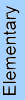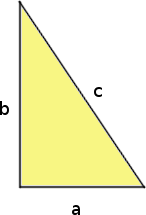## Posts

Showing posts from May, 2008

### HomeschoolMath.net new designMy main math site, Homeschoolmath.net , has gotten a face-lift... with better NAVIGATION on the left sidebar. Hopefully you'll be able to find your way around better along all the articles, reviews, worksheets, and stuff there. I especially want to draw attention to the ONLINE RESOURCES section. I used to have this on 9 pages... now I split it into 29 different pages, according to topic. These pages feature the best online games, quizzes, tutorials, or websites on various math-related topics. Have your pick: Online math resources menu Addition and subtraction Place value Clock Money Measuring Multiplication Division Math facts practice The four operations Factoring and number theory Geometry Fractions Decimals Percent Integers Statistics, Graphs, and Probability Geometry Algebra Calculus Trigonometry Logic and proof Problem solving & math projects For gifted children Math history Math games and fun websites Sites with interactive math tutoria

### I will derive!

Just a fun little song (parody of "I will survive") for all of us who've taken calculus.

### Heart of the Matter conference### Marbles problem to solve

Here's a nice "brain teaser" problem sent to my way... probably a student who wants to know the solution. I'll post it for you all and the solution in a few days. 204 marbles are divided into 3 groups according to colour. Ahmad found that there are twice as many blue marbles as white marbles and there are fewer red marbles than blue marbles. Ben found that the number of marbles in each group are divisible by 4 and 6. Cally found that the number of marbles in each group is less than 100. How many red marbles are there? (I do not have a clue where the problem originates, but I DO LIKE it!) Solution: Now, we need to find three numbers. The one hint tells us that one number is double the other, and the third is less than the first (the doubled one). This won't yet get us started. It is actually the latter hints that provide a starting point. We learn the numbers are divisible by 4. They're also divisible by 6, which means they're divisible by 2 and 3

### Saxon Math is not for everyone

(Updated in 2018) People sometimes ask me of my opinion or review of Saxon math. What I've written here applies in particular to Saxon Math's high school courses and middle grade levels. (The grades K-3 are by a different author and are quite different; more on that below.) Saxon Math uses an "incremental approach" where math concepts are studied in little pieces over several lessons, and those lessons are strawed over a long period of time, intermixed with lessons about other topics. In other words, if one lesson is on some particular topic (say, percentages or inequalities), it's almost guaranteed that the NEXT lesson is NOT on that topic . It jumps around from topic to topic constantly, and this is by design. Saxon's method also includes a feature where after a lesson is taught, there are very few practice problems about the topic of the lesson. Most of the problems are mixed review problems, and they practice concepts from earlier lessons, not th

### Teacher appreciation week

I should have posted this earlier but forgot. I even missed one day! Learning A-Z is having an open house this week; you can access their family of websites for free, one site per day. The writing site is for today. Vocabulary site is coming up soon, and so is their science site. Lots to explore and download.

### Gas price math

Today I just stumbled upon two sources discussing the price of gas in a comparative sense; one was a line graph comparing it to the past , the other was a world-wide comparison . Both were interesting; and a resourceful teacher can now make all kinds of problems based on the data. First based on the list of gas prices in various countries , for example these come to mind. (And I'm just giving you ideas for a lesson on gasoline lesson; I'm not providing answers but if some of you want to, feel free to comment.) Approximately how many-fold is the price of gas in Bosnia-Herzegovina as it is in the USA? In Egypt? In Venezuela? If your mileage is 25 miles per gallon, find the price of driving a 120-mile trip in Germany and in the USA. Looking at the inflation adjusted gas price in 2008 dollars now . Just reading the graph: When was the price of gas at its lowest? At its highest? How much was it? Find the price of gas (approximately) in 1930, 1960, and 1990 by reading the g

### A simple triangle problemSomeone sent in this very simple question (a student?). Leg b of the right triangle is twice as long as the base a . If the area is 36 cm squared, what is the length in of the leg b ? A little bit of algebra helps in this problem. FIRST strive to make a picture. Need a right triangle, the leg twice as long as the base. Here in my picture things aren't exactly to the scale, but it suffices for illustration purposes: So we actually know that b = 2a. The area of a triangle here is base times height over 2, and remember the height is the other leg, and it's twice the base: area = ba/2 = (2a)(a)/2 , and this is 36 (given). So we get our equation: (2a)(a)/2 = 36 a 2 = 36 a = 6. The leg b is therefore 12 cm long. check: Legs are 12 and 6, so the area is 12 * 6 / 2 = 36.